The critical concentrations of metal-SiO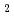and -HO mixtures were calculated for 26 actinides including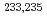U,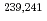Pu,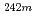Am,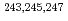Cm and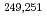Cf, where the critical concentration was defined as the concentration that the infinite neutron multiplication factor, k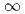being calculated to be 1.0. The calculations were performed using the Monte Carlo neutron transport calculation code MCNP5 combined with the evaluated nuclear data library JENDL3.3. The results showed that the critical actinide concentration of metal-SiOwas ca. 1/5 of that of metal-HO for all the fissile nuclides investigated. The k's of the metal-SiOand metal-HO at a half of the respective critical actinide concentration, which concentration was assumed as the subcritical actinide concentration limit, were found to be less than 0.8 for all the actinides considered. Applying a sum-of-fractions rule with respect to the ratios of actinide concentration to the subcritical actinide concentration limit for six fissile nuclides, subcriticality of high-level radioactive wastes was confirmed for a reported sample. The effect of different nuclear data libraries on the results of critical actinide concentrations was found large for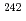Cm,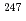Cm and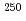Cf.